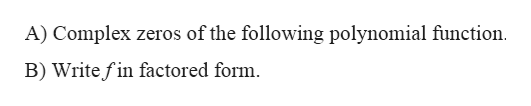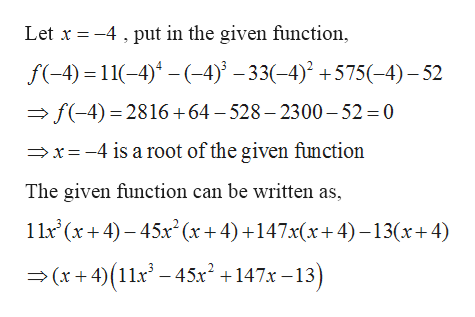Find the complex zeros of the following polynomial function.Write f in factored form. The complex zeros of f are  11x^4 - 1x^3 - 33x^2 + 575x - 52

Question
Find the complex zeros of the following polynomial function.
Write f in factored form.

The complex zeros of f are

11x^4 - 1x^3 - 33x^2 + 575x - 52
Step 1

Given: -

Step 2

To find: -help_outlineImage TranscriptioncloseA) Complex zeros of the following polynomial function B) Write fin factored form fullscreen
Step 3

Calculation...help_outlineImage Transcriptioncloseput in the given function, Let x4 f-4)11()4)^-33(4) +575(-4)-52 f(-4) 2816+64 - 528 - 2300-52 = 0 x-4 is a root of the given function The given function can be written as 1 1x (x4)45x(x+ 4)+147x(x+4)-13(x+4) (x+4)(11x-45x+147x-13) fullscreen

Want to see the full answer?

See Solution

Want to see this answer and more?

Our solutions are written by experts, many with advanced degrees, and available 24/7

See Solution
Tagged in

Other